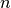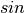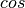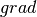# Need for a faster array¶

We know how lists work in Python. We also know that lists can hold the data items of various data types. This means that the list storage allocated to elements can vary in size. This factor makes the array access slow, and operations on array could take long time. numpy provides a elagent solution in the form of ndarray, a- Dimensional collections of elements with same data types. numpy also provides easier way to manipulate arrays, This makes it High Performance Numerical Calculation possible.

## Importing numpy¶

Following is the standard statement to import numpy. In future examples and library usages, we assume that you have imported the library in this way

In :

import numpy as np


## Creating ndarray from Lists¶

numpy allows us to create an array from exsisting Python List. Datatype conversions are performed if the input list contains elements of multiple datatypes. Datatypes are always promoted. Let’s look at some examples.

In :

a = np.array([1,2,3,4])
a

Out:

array([1, 2, 3, 4])

In :

b = np.array([,,])
b

Out:

array([,
,
])

In :

c = np.array([1,2,'x'])
c

Out:

array(['1', '2', 'x'],
dtype='<U21')


Note how int is converted into str. U21 is 32-bit Unicode Encoding. (Actual bits needed to store data is 21)

In :

d = np.array([1.3,1,3])
d

Out:

array([ 1.3,  1. ,  3. ])


Note how int is converted to float

## Accessing array elements and random shuffling¶

Array elements can be accessed using indices, slices and using masked arrays

Let’s create a random array and then illustrate the methods of accessing array elements

In :

x = np.arange(20)  # Like range(), but returns ndarry instead
x

Out:

array([ 0,  1,  2,  3,  4,  5,  6,  7,  8,  9, 10, 11, 12, 13, 14, 15, 16,
17, 18, 19])

In :

x.shape # (rows,cols)

Out:

(20,)

In :

x.shape = (4,5) # 4 rows 5 cols
x

Out:

array([[ 0,  1,  2,  3,  4],
[ 5,  6,  7,  8,  9],
[10, 11, 12, 13, 14],
[15, 16, 17, 18, 19]])

In :

x.size  # Total number of elements

Out:

20

In :

np.random.shuffle(x) # shuffles ndarray in-place
x

Out:

array([[15, 16, 17, 18, 19],
[ 5,  6,  7,  8,  9],
[10, 11, 12, 13, 14],
[ 0,  1,  2,  3,  4]])


This is how we can shuffle an array. random.shuffle() function takes an ndarray as an argument and sorts it in place. NEVER treat its return value as result!

In :

x

Out:

array([15, 16, 17, 18, 19])

In :

x  # Ok, inefficient

Out:

17


Above method is inefficient access because, it fetches x first and accesses it’s element at index 2. Next method computes the address from 2 co-ordinates directly, and fetches the element at one access

In :

x[0,2]   # Efficient

Out:

17

In :

x[0,1:4]

Out:

array([16, 17, 18])


Above example selects the elements at indices (0,1),(0,2),(0,3). Note that the slices can also be used to select elements from multi-dimensional array

In :

x[1:4,0]

Out:

array([ 5, 10,  0])

In :

x > 15

Out:

array([[False,  True,  True,  True,  True],
[False, False, False, False, False],
[False, False, False, False, False],
[False, False, False, False, False]], dtype=bool)


Note that it returned a boolean array after performing suitable operation. It is called masked array

In :

x [ x > 15 ]

Out:

array([16, 17, 18, 19])


This method to access array element is called as Access by Masked array

## Functions that operates on ndarrays¶

Numpy provides many Mathematical functions, that not only operates on inividual numbers, but also on entire arrays. Let’s illustrate them

In :

np.sin(x)

Out:

array([[ 0.65028784, -0.28790332, -0.96139749, -0.75098725,  0.14987721],
[-0.95892427, -0.2794155 ,  0.6569866 ,  0.98935825,  0.41211849],
[-0.54402111, -0.99999021, -0.53657292,  0.42016704,  0.99060736],
[ 0.        ,  0.84147098,  0.90929743,  0.14112001, -0.7568025 ]])

In :

x[np.sin(x) > 0] # elements whose sine is non-negative

Out:

array([15, 19,  7,  8,  9, 13, 14,  1,  2,  3])


many trigonometrical functions like,, calculus related functions likeare also available

### concatenate the arrays¶

concatenate((a1, a2, ...), axis=0) Join a sequence of arrays along an existing axis.

Parameters: - a1, a2, … : sequence of array_like

• The arrays must have the same shape, except in the dimension corresponding to axis (the first, by default).
• axis : int, optional
• The axis along which the arrays will be joined. Default is 0.
• Returns:
• res : ndarray
• The concatenated array.
• hstack((a1, a2, ...)) combines a1, a2, … horizontally
• vstack((a1, a2, ...)) combines a1, a2, … vertically
• dstack((a1, a2, ...)) combines a1, a2, … depthwise
In :

a = np.array([1,2,3,4])
b = np.array([9,8,7,6])

In :

a

Out:

array([1, 2, 3, 4])

In :

b

Out:

array([9, 8, 7, 6])

In :

np.concatenate((a,b),axis=0) # (a,b) is a tuple of arrays

Out:

array([1, 2, 3, 4, 9, 8, 7, 6])

In :

np.dstack((a,b))

Out:

array([[[1, 9],
[2, 8],
[3, 7],
[4, 6]]])

In :

np.vstack((a,b))

Out:

array([[1, 2, 3, 4],
[9, 8, 7, 6]])

In :

np.hstack((a,b))

Out:

array([1, 2, 3, 4, 9, 8, 7, 6])


We will use these functions frequently in upcoming chapters

### Aggregate Functions¶

Aggregate Functions are those which operates on entire array, to provide an overview of the elements

sum, average like functions fall in this catagory

We will use the below array to illustrate the usage of aggregate functions

In :

s = np.sin(x)
s

Out:

array([[ 0.        ,  0.84147098,  0.90929743,  0.14112001, -0.7568025 ],
[-0.95892427, -0.2794155 ,  0.6569866 ,  0.98935825,  0.41211849],
[-0.54402111, -0.99999021, -0.53657292,  0.42016704,  0.99060736],
[ 0.65028784, -0.28790332, -0.96139749, -0.75098725,  0.14987721]])

In :

np.sum(s) # You understood it, right?!

Out:

0.085276633692154075

In :

np.average(s)

Out:

0.0042638316846077039

In :

np.min(x)

Out:

0

In :

np.max(s)

Out:

0.99060735569487035


At current point, we will stop. This basic understanding of numpy is enough to understand the concepts of Algorithm Analysis in upcoming part.

Interested readers can refer the NumPy Official Tutorial at SciPy